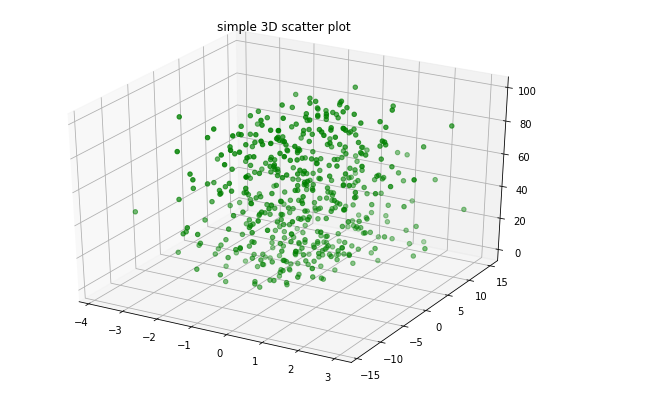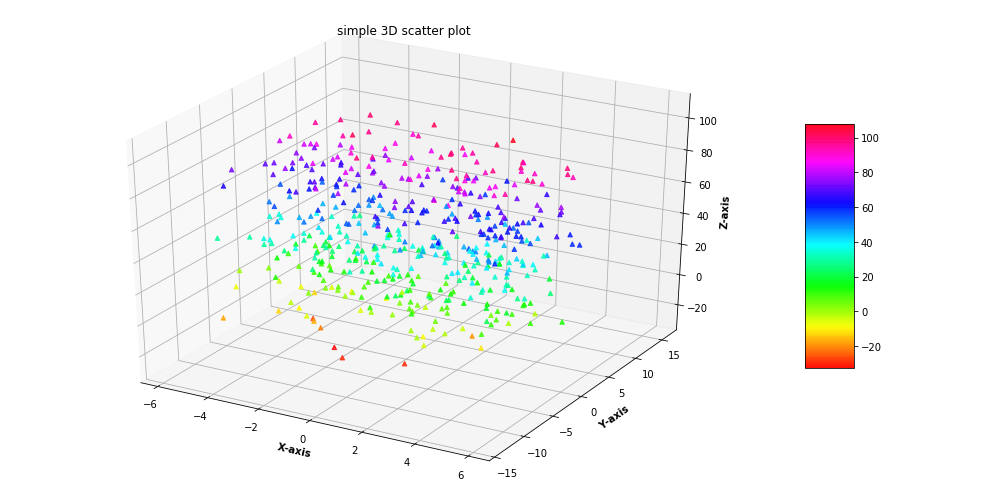Related Articles

# 3D Scatter Plotting in Python using Matplotlib

• Last Updated : 28 Sep, 2020

A 3D Scatter Plot is a mathematical diagram, the most basic version of three-dimensional plotting used to display the properties of data as three variables of a dataset using the cartesian coordinates.To create a 3D Scatter plot, Matplotlib’s mplot3d toolkit is used to enable three dimensional plotting.Generally 3D scatter plot is created by using ax.scatter3D() the function of the matplotlib library which accepts a data sets of X, Y and Z to create the plot while the rest of the attributes of the function are the same as that of two dimensional scatter plot.
Example 1: Let’s create a basic 3D scatter plot using the ax.scatter3D() function.

## Python3

 `# Import libraries` `from` `mpl_toolkits ``import` `mplot3d` `import` `numpy as np` `import` `matplotlib.pyplot as plt`     `# Creating dataset` `z ``=` `np.random.randint(``100``, size ``=``(``50``))` `x ``=` `np.random.randint(``80``, size ``=``(``50``))` `y ``=` `np.random.randint(``60``, size ``=``(``50``))`   `# Creating figure` `fig ``=` `plt.figure(figsize ``=` `(``10``, ``7``))` `ax ``=` `plt.axes(projection ``=``"3d"``)`   `# Creating plot` `ax.scatter3D(x, y, z, color ``=` `"green"``)` `plt.title(``"simple 3D scatter plot"``)`   `# show plot` `plt.show()`

Output :Example 2 : For better understanding Let’s take another example.

## Python3

 `# Import libraries` `from` `mpl_toolkits ``import` `mplot3d` `import` `numpy as np` `import` `matplotlib.pyplot as plt`     `# Creating dataset` `z ``=` `4` `*` `np.tan(np.random.randint(``10``, size ``=``(``500``))) ``+` `np.random.randint(``100``, size ``=``(``500``))` `x ``=` `4` `*` `np.cos(z) ``+` `np.random.normal(size ``=` `500``)` `y ``=` `4` `*` `np.sin(z) ``+` `4` `*` `np.random.normal(size ``=` `500``)`   `# Creating figure` `fig ``=` `plt.figure(figsize ``=` `(``16``, ``9``))` `ax ``=` `plt.axes(projection ``=``"3d"``)` `  `  `# Add x, y gridlines ` `ax.grid(b ``=` `True``, color ``=``'grey'``, ` `        ``linestyle ``=``'-.'``, linewidth ``=` `0.3``, ` `        ``alpha ``=` `0.2``) `     `# Creating color map` `my_cmap ``=` `plt.get_cmap(``'hsv'``)`   `# Creating plot` `sctt ``=` `ax.scatter3D(x, y, z,` `                    ``alpha ``=` `0.8``,` `                    ``c ``=` `(x ``+` `y ``+` `z), ` `                    ``cmap ``=` `my_cmap, ` `                    ``marker ``=``'^'``)`   `plt.title(``"simple 3D scatter plot"``)` `ax.set_xlabel(``'X-axis'``, fontweight ``=``'bold'``) ` `ax.set_ylabel(``'Y-axis'``, fontweight ``=``'bold'``) ` `ax.set_zlabel(``'Z-axis'``, fontweight ``=``'bold'``)` `fig.colorbar(sctt, ax ``=` `ax, shrink ``=` `0.5``, aspect ``=` `5``)`   `# show plot` `plt.show()`

Output :Attention geek! Strengthen your foundations with the Python Programming Foundation Course and learn the basics.

To begin with, your interview preparations Enhance your Data Structures concepts with the Python DS Course. And to begin with your Machine Learning Journey, join the Machine Learning – Basic Level Course

My Personal Notes arrow_drop_up
Recommended Articles
Page :